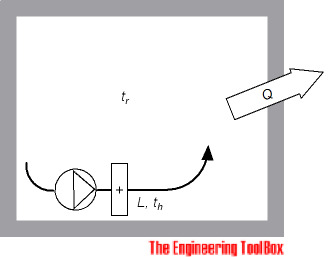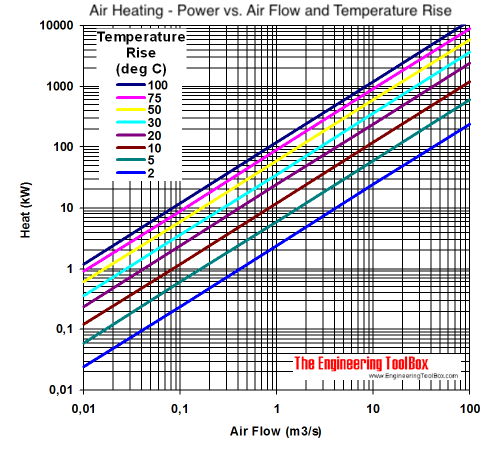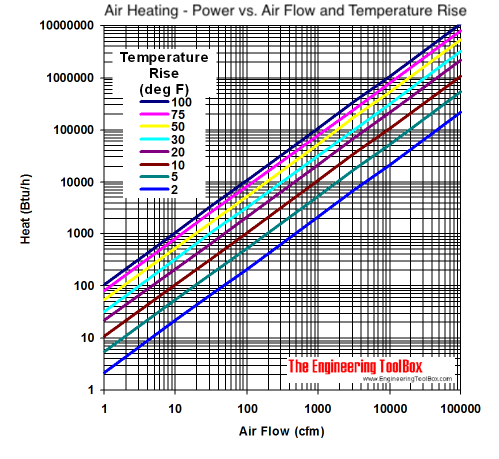Engineering ToolBox - Resources, Tools and Basic Information for Engineering and Design of Technical Applications!

# Air Heating Systems

## Air heating buildings - heat supply vs. air flow and temperature.

Air heating systems may be cost effective if they can be made simple or if they can be combined with a ventilation system. But - be aware that due to the low specific heat of air the use of air for heating purposes is very limited. Larger heat loads requires large volumes of air resulting in huge oversized ducts and fans. Transport of huge volumes of air requires a lot of energy.

### Required Air Volume in an Air Heating SystemRequired air flow rate in an air heating system can be calculated as

L = Q / (cp ρ (th - tr))                                         (1)

where

L = air flow rate (m3/s)

Q = heat loss covered by the air heating system (kW)

cp = specific heat air - 1.005 (kJ/kgoC)

ρ = density of air - 1.2 (kg/m3)

th = heating air temperature (oC)

tr = room temperature (oC)

As a rule of thumb the air heating supply temperature should be in the range 40-50oC. The air flow should be in the range 1-3 times the room volume.

Equation (1) expressed in imperial units:

L = Q / (1.08 (th - tr))                                        (2)

where

Q = heat (btu/hr)

L = air volume (cfm)

th = heating air temperature (oF)

tr = room temperature (oF)

### Online Air Heating Calculator

Q = heat loss from the building (kW)

ρ = density of air - 1.2 (kg/m3)

th = heating air temperature (oC)

tr = room temperature (oC)

### Air Heating - Temperature Rise Diagram

The diagrams below are calculated from the equations above and can be used to estimate heat required to rise temperature in air flows.

#### SI units - kW, m3/s and oC#### Imperial units - Btu/h, cfm and oF• 1 m3/s = 3,600 m3/h = 35.32 ft3/s = 2,118.9 ft3/min (cfm)
• 1 kW (kJ/s) = 859.9 kcal/h = 3,413 Btu/h
• T(oC) = 5/9[T(oF) - 32]

#### Example - Heating a single room with air

A building with a large room with a heat loss 20 kW is heated with air with a maximum temperature 50 oC. The room temperature is 20 oC. The required air flow rate can be calculated as

L = (20 kW) / ((1.005 kJ/kgoC) (1.2 kg/m3) ((50 oC) - (20 oC)))

= 0.55 m3/s

### Required Air Flow from an Electric Furnace - Imperial Units

Required air flow from an electric furnace can be expressed in Imperial units as

Lcfm = Pw 3.42 / 1.08 dt                                   (3)

where

Lcfm = required air flow (cfm)

P= electric power (watts)

dt = temperature difference (oF)

## Related Topics

• Heating - Heating systems - capacity and design of boilers, pipelines, heat exchangers, expansion systems and more.
• Ventilation - Systems for ventilation and air handling - air change rates, ducts and pressure drops, charts and diagrams and more.

## Engineering ToolBox - SketchUp Extension - Online 3D modeling!

Add standard and customized parametric components - like flange beams, lumbers, piping, stairs and more - to your Sketchup model with the Engineering ToolBox - SketchUp Extension - enabled for use with the amazing, fun and free SketchUp Make and SketchUp Pro .Add the Engineering ToolBox extension to your SketchUp from the SketchUp Pro Sketchup Extension Warehouse!

Translate

## Privacy

We don't collect information from our users. Only emails and answers are saved in our archive. Cookies are only used in the browser to improve user experience.

Some of our calculators and applications let you save application data to your local computer. These applications will - due to browser restrictions - send data between your browser and our server. We don't save this data.

## Citation

• Engineering ToolBox, (2008). Air Heating Systems. [online] Available at: https://www.engineeringtoolbox.com/air-heating-systems-d_1136.html [Accessed Day Mo. Year].

Modify access date.

. .

#### Scientific Online Calculator6 24